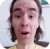FreeKB - Perl Convert hexadecimal to decimal
Perl - Convert hexadecimal to decimalDecimal and Hex

Decimal is what we typically thing of when we thing of numbers. Decimal is simply the numbers 0 1 2 3 4 5 6 7 8 9. Decimal is also known as base 10, because there are 10 unique numbers.

Hexadecimal contains the follow numbers and letters: 0 1 2 3 4 5 6 7 8 9 A B C D E F. Hexadecimal is also known as base 16, because there are 16 unique values.In Hexadecimal, A has a value of 10, B 11, C 12, D 13, E 14, and E 15.

The hex function can be used to convert hexadecimal to decimal. For example, the "f" character in hexadecimal is the number 15 in decimal. The following Perl script would convert f to 15.

``````#!/usr/bin/perl
print hex 'f';``````

Hexadecimal strings are often predeeded with 0x. Appending 0x before a hexadecimal string will not impact the conversion of the string to decimal.

``````#!/usr/bin/perl
print hex '0xf';``````

Hex can just as well be used when reading or writing to a file.

``````\$file = "/path/to/file.txt";
open(FH, '<', \$file) or die "cannot open \$file \$! \n";

while (<FH>) {
print hex \$_;
}

close(FH);``````

Integer overflow

The hex function may retur "Integer overflow in hexadecimal number" when using large hexadecimal strings.

``````Integer overflow in hexadecimal number at example.pl line x.
2.95147905179353e+20``````

The Math::BigInt module may be able to convert large hexadecimal strings to decimal.

``````#!/usr/bin/perl
use Math::BigInt;

\$x = Math::BigInt->new("0xffffffffffffffffffffffff");
print \$x;``````

We will never share your name or email with anyone. Enter your email if you would like to be notified when we respond to your comment.

Please enter a5aa8 in the box below so that we can be sure you are a human.

Web design by yours truely - me, myself, and I   |   jeremy.canfield@freekb.net   |